# Analysis Of Binomial Heaps Operations Insert Add a

• Slides: 18Analysis Of Binomial Heaps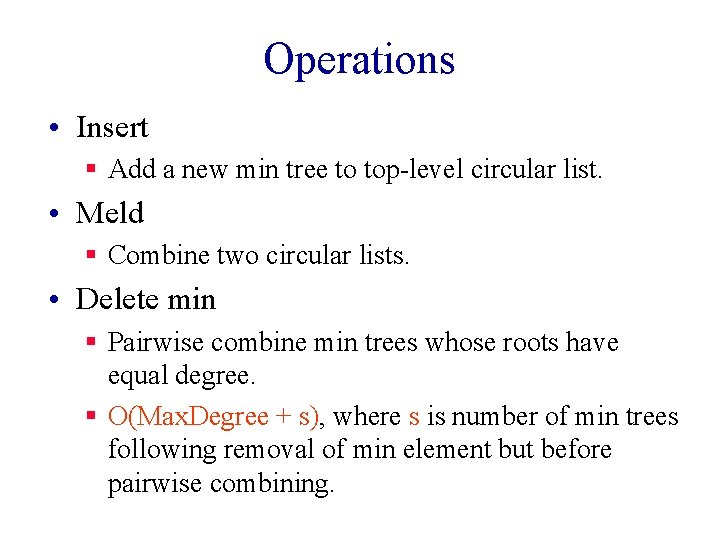Operations • Insert § Add a new min tree to top-level circular list. • Meld § Combine two circular lists. • Delete min § Pairwise combine min trees whose roots have equal degree. § O(Max. Degree + s), where s is number of min trees following removal of min element but before pairwise combining.Binomial Trees • Bk , k > 0, is two Bk-1 s. • One of these is a subtree of the other. B 0 B 1 B 2 B 3All Trees In Binomial Heap Are Binomial Trees • Insert creates a B 0. • Meld does not create new trees. • Pairwise combine takes two trees of equal degree and makes one a subtree of the other. • Let n be the number of operations performed. § § Number of inserts is at most n. No binomial tree has more than n elements. Max. Degree <= log 2 n. Complexity of remove min is O(log n + s) = O(n).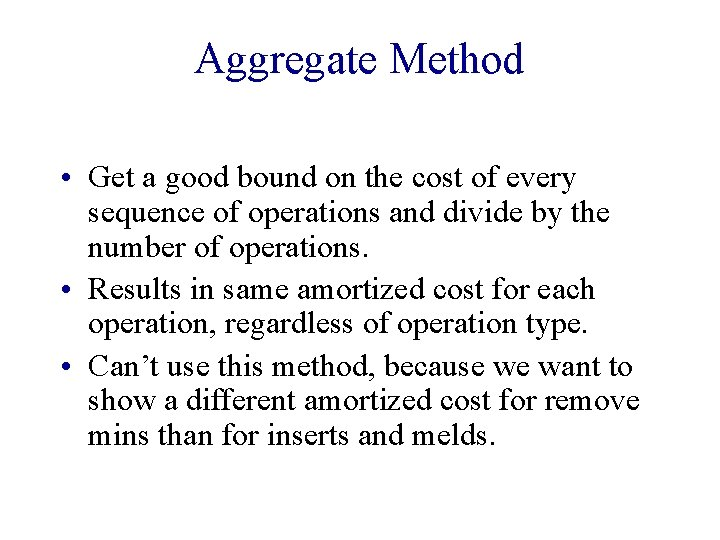Aggregate Method • Get a good bound on the cost of every sequence of operations and divide by the number of operations. • Results in same amortized cost for each operation, regardless of operation type. • Can’t use this method, because we want to show a different amortized cost for remove mins than for inserts and melds.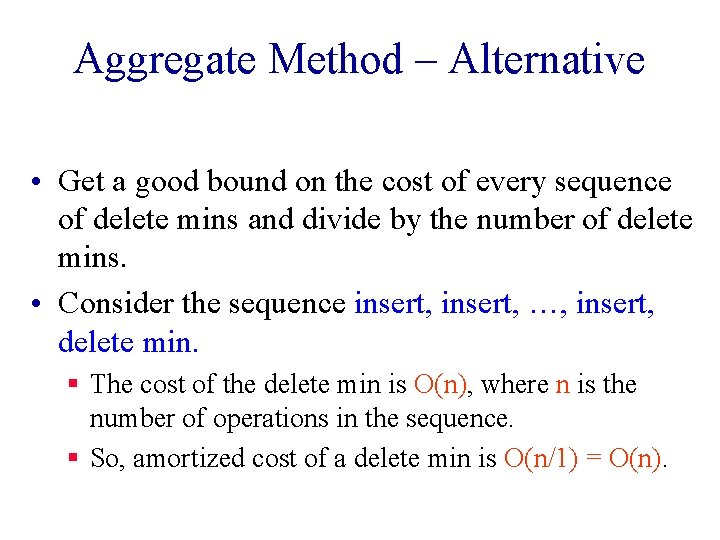Aggregate Method – Alternative • Get a good bound on the cost of every sequence of delete mins and divide by the number of delete mins. • Consider the sequence insert, …, insert, delete min. § The cost of the delete min is O(n), where n is the number of operations in the sequence. § So, amortized cost of a delete min is O(n/1) = O(n).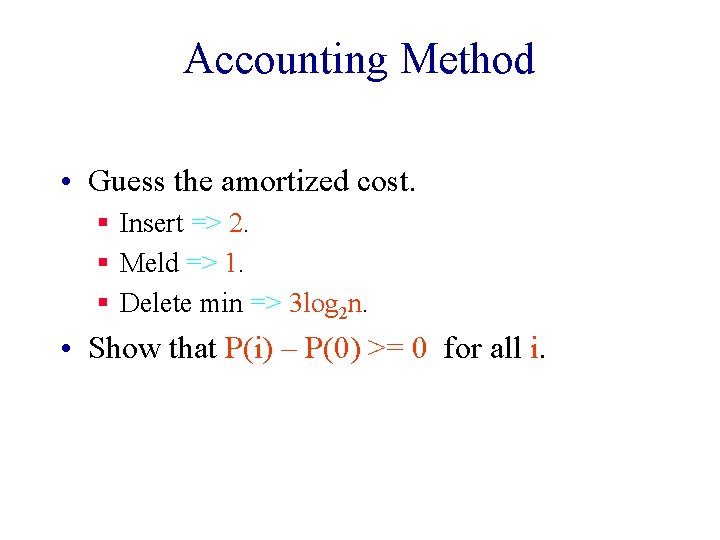Accounting Method • Guess the amortized cost. § Insert => 2. § Meld => 1. § Delete min => 3 log 2 n. • Show that P(i) – P(0) >= 0 for all i.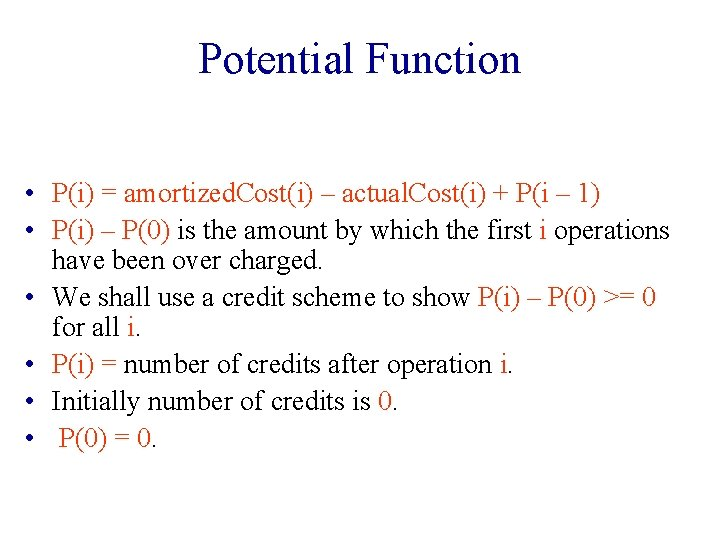Potential Function • P(i) = amortized. Cost(i) – actual. Cost(i) + P(i – 1) • P(i) – P(0) is the amount by which the first i operations have been over charged. • We shall use a credit scheme to show P(i) – P(0) >= 0 for all i. • P(i) = number of credits after operation i. • Initially number of credits is 0. • P(0) = 0.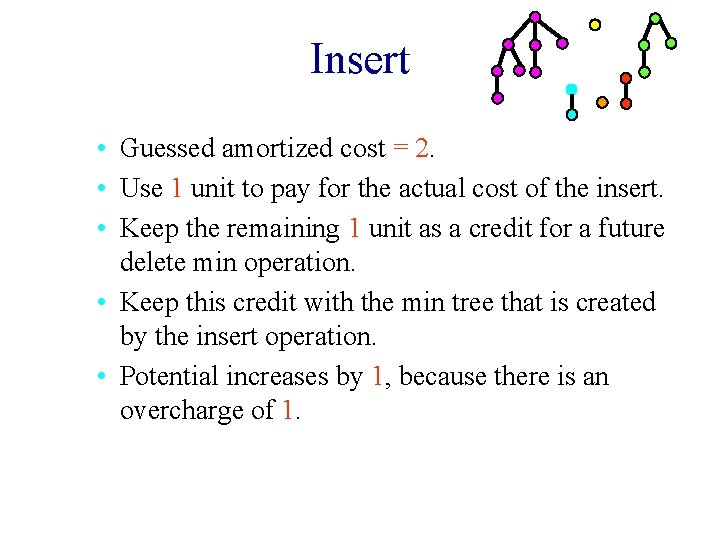Insert • Guessed amortized cost = 2. • Use 1 unit to pay for the actual cost of the insert. • Keep the remaining 1 unit as a credit for a future delete min operation. • Keep this credit with the min tree that is created by the insert operation. • Potential increases by 1, because there is an overcharge of 1.Meld • Guessed amortized cost = 1. • Use 1 unit to pay for the actual cost of the meld. • Potential is unchanged, because actual and amortized costs are the same.Delete Min • Let Min. Trees be the set of min trees in the binomial heap just before delete min. • Let u be the degree of min tree whose root is removed. • Let s be the number of min trees in binomial heap just before pairwise combining. § s = #Min. Trees + u – 1 • Actual cost of delete min is <= Max. Degree + s <= 2 log 2 n – 1+ #Min. Trees.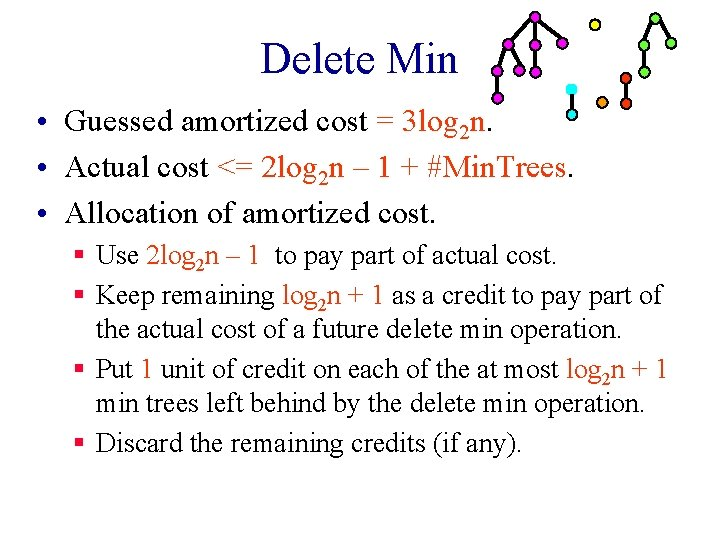Delete Min • Guessed amortized cost = 3 log 2 n. • Actual cost <= 2 log 2 n – 1 + #Min. Trees. • Allocation of amortized cost. § Use 2 log 2 n – 1 to pay part of actual cost. § Keep remaining log 2 n + 1 as a credit to pay part of the actual cost of a future delete min operation. § Put 1 unit of credit on each of the at most log 2 n + 1 min trees left behind by the delete min operation. § Discard the remaining credits (if any).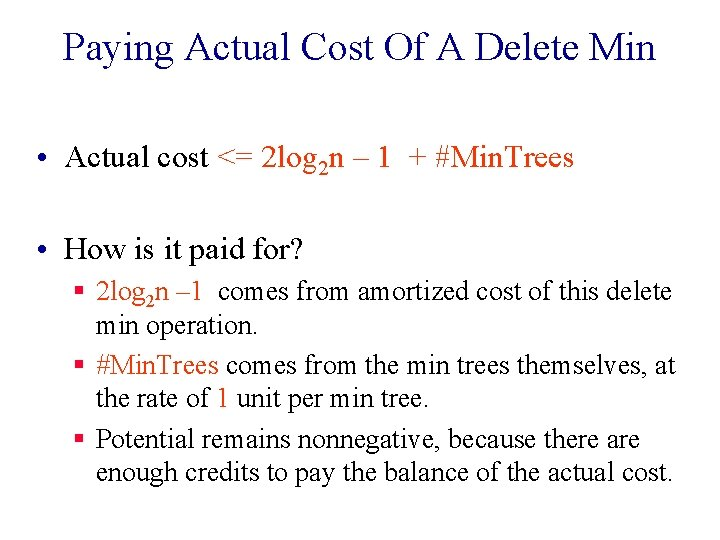Paying Actual Cost Of A Delete Min • Actual cost <= 2 log 2 n – 1 + #Min. Trees • How is it paid for? § 2 log 2 n – 1 comes from amortized cost of this delete min operation. § #Min. Trees comes from the min trees themselves, at the rate of 1 unit per min tree. § Potential remains nonnegative, because there are enough credits to pay the balance of the actual cost.Potential Method • Guess a suitable potential function for which P(i) – P(0) >= 0 for all i. • Derive amortized cost of ith operation using DP = P(i) – P(i – 1) = amortized cost – actual cost • amortized cost = actual cost + DP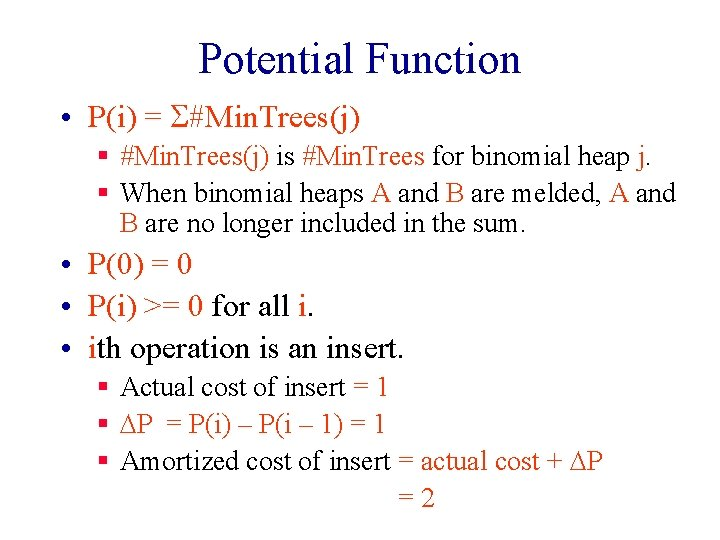Potential Function • P(i) = S#Min. Trees(j) § #Min. Trees(j) is #Min. Trees for binomial heap j. § When binomial heaps A and B are melded, A and B are no longer included in the sum. • P(0) = 0 • P(i) >= 0 for all i. • ith operation is an insert. § Actual cost of insert = 1 § DP = P(i) – P(i – 1) = 1 § Amortized cost of insert = actual cost + DP =2ith Operation Is A Meld • Actual cost of meld = 1 • P(i) = S#Min. Trees(j) · DP = P(i) – P(i – 1) = 0 • Amortized cost of meld = actual cost + DP =1ith Operation Is A Delete Min • old => value just before the delete min • new => value just after the delete min. • #Min. Treesold(j) => value of #Min. Trees in jth binomial heap just before this delete min. • Assume delete min is done in kth binomial heap.ith Operation Is A Delete Min • Actual cost of delete min from binomial heap k <= 2 log 2 n – 1 + #Min. Treesold(k) · DP = P(i) – P(i – 1) = S[#Min. Treesnew(j) – #Min. Treesold(j)] = #Min. Treesnew(k) – #Min. Treesold(k). • Amortized cost of delete min = actual cost + DP <= 2 log 2 n – 1 + #Min. Treesnew (k) <= 3 log 2 n.